• EN

# Handy Instruction Manual

## 26Using Variables

The following will explain how to use variables to store and calculate numerical values.

### Assigning a Value to a Variable with the = Sign

Putting something (a value) inside a variable is called "assignment."In this figure, the value 5 is assigned to variable A.
In BASIC, this is written as follows: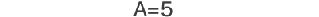This doesn't mean "A is equal to 5," but is an instruction meaning "assign 5 to A." If variable A does not exist yet, an empty piece of memory will be created and given the name A.

Let's try using the PRINT instruction to check if the 5 really was assigned to variable A.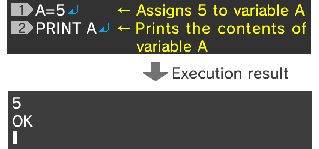#### ◆Character strings cannot be assigned to numerical variables

To be precise, the variable described here is called a "numerical variable." You cannot assign character strings to this type of variable. For example, inputting A="HELLO" will cause an error.
To assign a character string to a variable, you need to use a "string variable," which will be described later.

#### ◆Formulas are also allowed

As well as single values, you can also write formulas to the right of the = sign. However, you need to use an asterisk (*) as the multiplication sign, and a slash (/) as the division sign.

Let's prepare two variables, A and B, and assign to them the calculation results of "2+3" and "3÷2" respectively.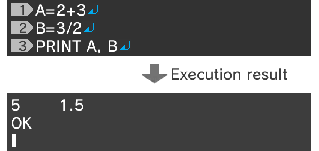#### ◆Calculations between variables

You can also perform calculations between variables, as well as between numerical values.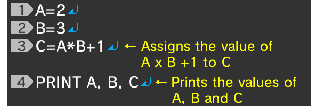In the third line, the content of A is multiplied by the content of B, 1 is added, and the result is assigned to C.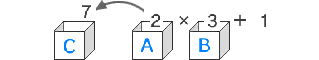The execution result will be as follows: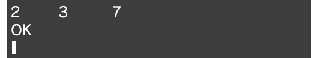#### ◆Find the area of a circle

Let's try writing a program to calculate the area of a circle with a radius of 2.

The area of a circle is radius x radius x pi. Here, let's use 3.14 as pi.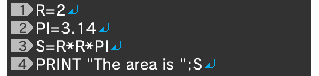In the second line, the decimal number 3.14 is assigned to the variable PI. Variable names do not have to be a single character. You can use names with any length you wish, as long as they begin with a letter character and consist only of alphanumeric characters and underscores (_).

In the third line, the area is calculated, and assigned to the variable S.

Pay attention to the PRINT instruction in the fourth line. This instruction prints "The area is " first, and then directly after that, as specified by the semicolon (;), prints the calculation result S.

The execution result will be as follows: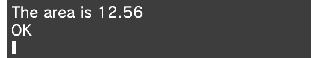### Character Strings and String Variables

To assign a character string to a variable, you need to use what's called a "string variable."
String variable names should have a \$ sign at the end.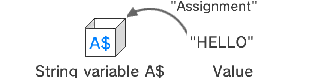In BASIC, this is written as follows: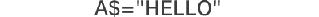You can use the PRINT instruction to print the value of a string variable in the same way as that of a numerical variable. The following program assigns "ALICE" to the string variable NAME\$, and then uses the PRINT instruction to print the string.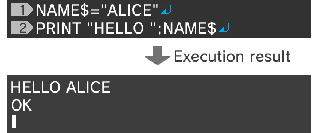You can use addition to join string variables.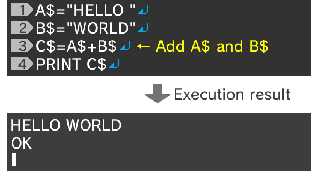PDF File

e-manual.pdf (3.0 MB)

• 1

• 2345678